Electron. J. Diff. Eqns. Vol. 1997(1997), No. 05, pp 1-11.

### On Neumann boundary value problems for some quasilinear elliptic equations Paul A. Binding, Pavel Drabek, & Yin Xi Huang

Abstract:
We study the role played by the indefinite weight function a(x) on the existence of positive solutions to the problem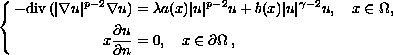where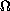is a smooth bounded domain in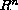, b changes sign,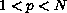,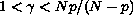and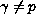. We prove that

1. if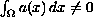and b satisfies another integral condition, then there exists some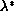such that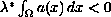and, for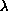strictly between 0 and, the problem has a positive solution.
2. if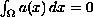, then the problem has a positive solution for smallprovided that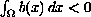.

Submitted September 11, 1996. Published January 30, 1997.
Math Subject Classification: 35J65, 35J70, 35P30.
Key Words: p-Laplacian, positive solutions, Neumann boundary value problems.

Show me the PDF file (182 KB), TEX file, and other files for this article.
 Paul A. Binding Department of Mathematics & Statistics, University of Calgary, Calgary, Alberta, Canada, T2N 1N4 e-mail: binding@acs.ucalgary.caPavel Drabek Department of Mathematics University of West Bohemia P.O. Box 314, 30614 Pilsen, Czech Republic e-mail: pdrabek@kma.zcu.cz http://deimos.zcu.cz/Drabek Yin Xi Huang Department of Mathematical Sciences, University of Memphis, Memphis, TN 38152 USA e-mail: huangy@mathsci.msci.memphis.edu